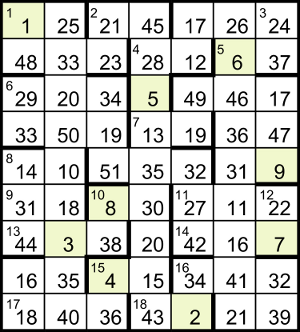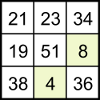## Magic Cuboid by Brimstone

### Puzzle solution process

This is one possible solution path. We’ll use commas to show how numbers are split into the available cells; for example, a three-digit number abc could be entered in two cells as either a,bc or ab,c.

In 16ac, the square of the last term is F14. The three cells can hold a number of up to 6 digits, but 314 = 4782969 is too big, so F = 2.

Then the square of the last term in 6dn is 4×V6, for an entry of up to 4 digits; 56 = 15625 is too big, so V = 3. This provides the following values for grid entries: 18ac = 432, 1dn = 148, 6dn = 2933 (entered as 29,33) and 16dn = 342. 18ac and 16dn share the same last cell, so they’re entered as 43,2 and 34,2 respectively.

The last term of 6ac gives a square of 16×J6. If J is 7, that would be 1882384, too big for the 3 cells available, so J = 5. This provides the following values for grid entries: 1ac = 125, 10ac = 830 (entered as 8,30 because 83 and 0 aren’t valid cell contents), 12dn = 227 and 15dn = 436.

Since 1ac = 125 and 1dn = 148, they must be entered as 1,25 and 1,48 respectively. We now have the top three cells in the first column filled, so the total for rows and columns of the magic square must be 1+48+29 = 78. The 1st, 4th and 7th cells in grid column 1 form a column in a magic square, so 8dn must end in 78−1−33 = 44. The 3rd, 6th and 9th cells in grid column 4 form a column in a magic square, so the 3rd cell must contain 78−30−43 = 5. Similarly, the cells at the bottom of column 5 contain _,34,2 so 14ac starts with 78−34−2 = 42.

For 16ac we have 34,_,_ and the clue gives 324U2+32768; the smallest available prime is 7 but 324×72+32768 = 48644, so the entry must be a 6-digit number in the range [341010, 345151]. That puts U2 between (341010−32768)/324 ≈ 951.4 and (345151−32768)/324 ≈ 964.1, so U = 31 and 16ac = 34,41,32.

The first cell of 10dn contains 8 and the value is 4K2+162, so it must be a 3-digit number in the range [810, 851]. That puts K2 in the range (810−162)/4 = 162 to (851−162)/4 = 172.25, so K = 13 and 10dn = 8,38. Now 15dn can’t be entered as 43,6 because 38+43+6 = 87 doesn’t match the magic total of 78, so 15dn = 4,36. The 3rd, 6th and 9th cells in column 3 are now _,8,36, so to make the total of 78 the 3rd cell contains 34.

For 6ac the clue gives 25E2+250009 and the grid gives 29,_,34 so it must be a 6-digit number in the range [291034, 295134]. That puts E2 in the range (291034−250009)/25 = 1641 to (295134−250009)/25 = 1805, so E = 41 and 6ac = 29,20,34. The top 3 cells of column 2 are now 25,_,20, so they must be 25,33,20 to make a total of 78.

For 17ac the clue gives 36Q4+97600 and the entry has 3 cells. If Q is 11 the answer is 624676, which is too big, as 62 isn’t allowed in a cell. So Q = 7 and 17ac = 18,40,36. The 3rd, 6th and 9th cells in column 2 must add up to 78, so 9ac ends with 78−20−40 = 18. The 3rd, 6th and 9th cells in column 1 must add up to 78, so 9ac starts with 78−29−18 = 31. To make up the magic totals, the middle 3 cells of column 1 are 33,14,31 and the bottom 3 cells are 44,16,18.

The clue for 4ac now gives 28,12 which makes _,28,5 in the top 3 cells of column 4; to make 78 they must be 45,28,5. The clue for 7ac gives 13,19 which makes 13,_,30 in the top 3 cells of column 4; to make 78 they must be 13,35,30. Then column 4’s magic square can be completed with 20,15,43 in the bottom 3 cells.

In column 5 the 1st, 4th and 7th cells contain _,19,42 so the 1st cell must have 17. The 2nd, 5th and 8th cells contain 12,_,34 so the 5th must have 32. Then the column can be completed with 17,12,49 in the top 3 cells and 19,32,27 in the middle.

The clue for 8ac gives 14,10 which makes _,10,18 in the middle 3 cells of column 2; to make 78 they must be 50,10,18. Then column 2 can be completed with 3,35,40 in the bottom 3 cells. The clue for 2dn gives 21,23 and column 3 can be completed with 19,51,8 in the middle 3 cells.The clues give 5ac = 6,37 (not 63,7 because 63 isn’t allowed in a cell) and 3dn = 24,37. To make 78, the top 3 cells in column 7 must be 24,37,17. To complete totals in the 2dn, 5th and 8th cells in columns 6 and 7, the 5th row must end with 31,9.

The grid gives 3118 for 9ac = 9R2+2029, so R = 11. That makes 11ac = 27,11,22 so 12dn is entered as 22,7 rather than 2,27. The bottom cells in column 7 are now 7,32,_, so the bottom cell contains 78−7−32 = 39 and then the column can be completed with 47,9,22 in the middle cells. Similarly, the middle cells of column 6 are _,31,11 so they must be 36,31,11.

The grid gives 44338 for 13ac = (2M+7)2+14409, so M = 83 and 14ac = 42,16,7. Magic square totals now allow completion of column 6 with 16,41,21 at the bottom and 26,6,46 at the top. The initial grid is now complete.

Every 90-degree rotation of one of the magic squares has no effect on its central cell, so the 5th row of the grid (including the cell containing 9) will remain unchanged; the final total for all rows will therefore be 14+10+51+35+32+31+9 = 182. We’re told that 1 is to be in the top-left cell, so the 1st column won’t be rotated but all the rest will, so that all of the cells containing 2 to 8 change.Column 3 represents the magic square shown here. If it’s rotated once (anticlockwise), the new values in the 4 and 8 cells will be 19 and 4 respectively; twice gives 23 and 19; three times gives 8 and 23. The possible new values for all of the affected cells, classified as Prime, Square or Multiple of 4, are as follows:

Cell 1 rotation 2 rotations 3 rotations
2 (42) 17 (P) 49 (S)
3 25 (S) 20 (M) 40 (M)
4, 8 19 (P), 4 (S) 23 (P), 19 (P) 8 (M), 23 (P)
5 43 (P) 20 (M) (45)
6 11 (P) 41 (P) 36 (S)
7 24 (M) 17 (P) (39)

If (4, 8) → (23, 19) then both primes are used, so 2 → 49 and 6 → 36 (both squares). However, we’re aiming at a total of 200 from all nine cells, made up of (1+9) + (three multiples of 4) + (two odd primes) + (two squares); as the first three terms are all even, to get an even total the two squares must be either both odd or both even, so they can’t be 49 and 36.

If (4, 8) → (19, 4) then 2 and 6 must account for the other prime and square (because they have no multiples of 4) and as the second square must be even, 2 → 17 and 6 → 36. The rest must become multiples of 4, ie, 7 → 24, 5 → 20 and 3 → 40 (not 20, because then it would have the same value as the 5 cell). The total of the cells would then be 1+17+40+19+20+36+24+4+9 = 170, not 200 as specified.

Therefore (4, 8) → (8, 23). The only squares available are 2 → 49, 3 → 25, 6 → 36, but as they must be both odd or both even we can rule out 36. So 6 → 11 or 41 (prime), which means that the rest must be multiples of 4, ie, 5 → 20 and 7 → 24. If 6 → 11 the total is 1+49+25+8+20+11+24+23+9 = 170, so we must have 6 → 41 and 1+49+25+8+20+41+24+23+9 = 200. Making the required rotations, we can verify that all nine grid rows have cell totals of 182.